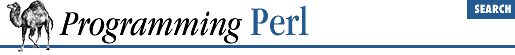Chapter 3Functions3.2.91 map

map BLOCK LIST
map EXPR, LIST

This function evaluates the BLOCK or EXPR for each element of LIST (locally setting \$_ to each element) and returns the list value composed of the results of each such evaluation. It evaluates BLOCK or EXPR in a list context, so each element of LIST may produce zero, one, or more elements in the returned value. These are all flattened into one list. For instance:

@words = map { split ' ' } @lines;

splits a list of lines into a list of words. Often, though, there is a one-to-one mapping between input values and output values:

@chars = map chr, @nums;

translates a list of numbers to the corresponding characters. And here's an example of a one-to-two mapping:

%hash = map { genkey(\$_), \$_ } @array;

which is just a funny functional way to write this:

%hash = ();
foreach \$_ (@array) {
\$hash{genkey(\$_)} = \$_;
}

See also grep. map differs from grep in that map returns a list consisting of the results of each successive evaluation of EXPR, whereas grep returns a list consisting of each value of LIST for which EXPR evaluates to true.